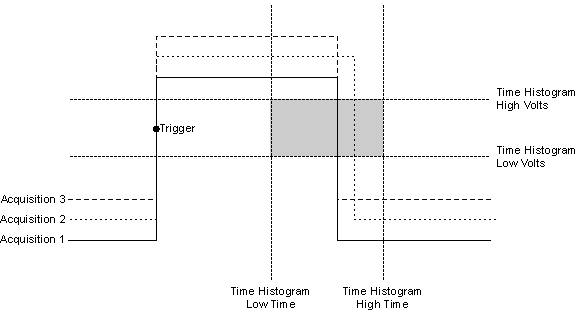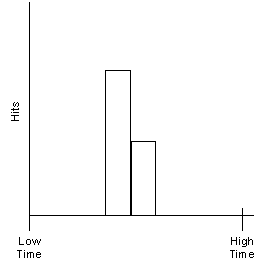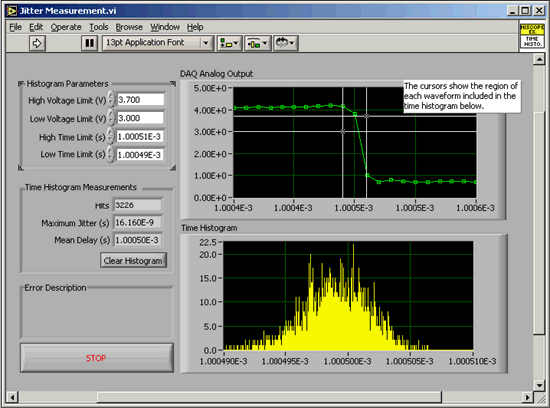# Time Histograms

NI High-Speed Digitizers Help (NI-SCOPE)

Edition Date: January 2017

Part Number: 370592AB-01

View Product InfoNI-SCOPE (English | Japanese)

Time histograms place samples that fall within a defined voltage range and time window into bins based on their time relative to a trigger point. The time resolution of time histograms depends directly on the precision with which samples can be positioned in time relative to the trigger. More precise positioning of the sample in time allows the use of more histogram bins of smaller size, increasing histogram resolution. Often, digitizers can only resolve samples in time with a resolution equal to the sample period. Many NI digitizers, however, include a high-resolution Time-to-Digital Conversion (TDC) circuit. Using a process called time-stamping, the TDC circuit locates samples relative to the trigger point with exceptional precision. For example, the NI 5122 is equipped with a TDC circuit that allows time-stamping of samples with 100 ps resolution, while using a 10 ns sample period. Thus, TDC circuitry and time-stamping allow you to sort data into highly resolved time histogram bins and maximize histogram resolution.

The following figures demonstrate how time histograms are constructed. The first figure is a time-domain waveform sampled by a high-speed digitizer. The second figure shows the corresponding time histogram. Multiple pulses are acquired, and an edge trigger is used to align the rising edge of each pulse. Histogram voltage (vertical) discriminator levels are configured to define a window around the falling edges of each pulse—the shaded region in the figure. Make sure you capture only the falling edges of the waveform when setting these discriminator levels—otherwise, the histogram appears perfectly uniform.When you use this setup, every sample on the falling edge is added to the time histogram shown in the following figure. In this example, both the first and third acquisitions have a falling edge at the same time, while the second acquisition is later. The histogram captures this statistical information.## Time Histogram Measurements Application

Histograms can be used to characterize delay jitter between the input and output of a real-time I/O system. By definition, a real-time system is deterministic in time, but it is useful to know exactly how deterministic a given measurement system is. Time histogram measurements can be used to characterize the jitter in real-time systems, which in turn allows measurement of the determinism of a real-time I/O system.

Let's look at a specific example: feeding a square wave into the analog input of a real-time system while sampling at a rate of 1 kHz. The samples acquired are output to an analog channel by the real-time system one clock cycle later. This provides a deterministic delay of 1 msec between the analog input and the analog output of the real-time system. Measurement of the jitter in this delay between the input and output yields a measure of the reliability of the real-time I/O system.

In this example, the NI 5122 two-channel high-speed digitizer is used to measure the jitter. The input square wave and the analog output of the real-time system are sampled by the NI 5122. A time histogram of the delay from the edge on one channel to the same edge on the second channel is created. Statistics from the time histogram such as Time Histogram Min, Time Histogram Max, Time Histogram Hits and Time Histogram Mean yield measurements of the maximum jitter, the number of measured samples in the histogram, and the mean delay.

The results shown in the following figure yield a Gaussian distribution of the variations of the delay, meaning that this jitter is most likely derived from random electrical noise in the measurement system.#### Histogram Measurements of Delay Between Analog Input and Analog Output on a Real-Time I/O System.Note  Maximum (peak-to-peak) jitter is approximately 16 ns for the Real-Time I/O system of 1 ms delay.